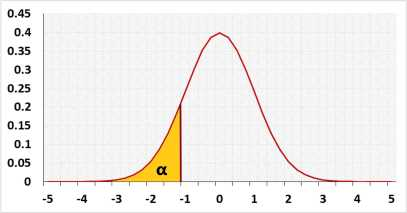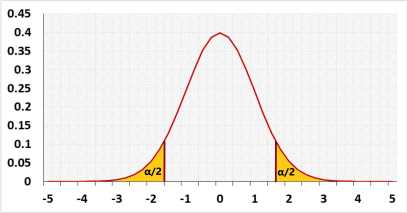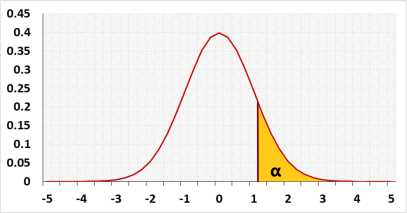# Correlation Coefficient Calculator

The Correlation Calculator computes both Pearson and Spearman's Rank correlation coefficients, and tests the significance of the results. Additionally, it calculates the covariance.
You may change the X and Y labels. Separate data by Enter or comma, , after each value. The tool ignores non-numeric cells.

## Correlation calculator

Calculates and test the correlation.

### What is covariance?

The covariance checks the relationship between two variables.
The covariance range is unlimited from negative infinity to positive infinity. For independent variables, the covariance is zero.
Positive covariance - changes go in the same direction, when one variable increases usually also the second variable increases, and when one variable decreases usually also the second variable decreases.
Negative covariance - opposite direction, when one variable increases usually the second variable decreases, and when one variable decreases usually the second variable increases.

#### How to calculate the covariance

The covariance formula is:
Cov(X,Y) = E[(X-E[X])(Y-E[Y])]
Cov(X,Y) = E[XY]-E(X)E[Y]
SXY - the sample covariance between X and Y.
 SXY = Σ(xi-x̄)(yi-ȳ) n - 1

### What is correlation?

You may say that there is a correlation between two variables, or statistical association, when the value of one variable may at least partially predict the value of the other variable.
The correlation is a standardized covariance, the correlation range is between -1 and 1.
The correlation ignores the cause and effect question, is X depends on Y or Y depends on X or both variables depend on the third variable Z.
Similarly to the covariance, for independent variables, the correlation is zero.
Positive correlation - changes go in the same direction, when one variable increases usually also the second variable increases, and when one variable decreases usually also the second variable decreases.
Negative correlation - opposite direction, when one variable increases usually the second variable decreases, and when one variable decreases usually the second variable increases.
Perfect correlation - When you know the value of one variable you may calculate the exact value of the second variable. For a perfect positive correlation r = 1. and for a perfect negative correlation r = -1.

### What is the Pearson correlation coefficient?

The Pearson correlation coefficient is a type of correlation, that measure linear association between two variables

#### How to calculate the Pearson correlation?

##### Population Pearson correlation formula
 ρXY = E[(X-E[X])(Y-E[Y])] σXσY
Population Pearson correlation formula - using the covariance
 ρ = Cov(X,Y) σXσY
##### Sample Pearson correlation formula
 r = Σ(xi - x̄)(yi - ȳ) √(Σ(xi - x̄)2Σ(yi - ȳ)2 )

Sample Pearson correlation formula - using the covariance
 r = SXY SXSY

#### Correlation effect size

The correlation value is also the correlation effect size.
Define the level of the effect size is only a rule of thumb. Following Cohen's guidelines (Cohen 1988 - pg 413)

Correlation value(r)Level
|r| < 0.1Very small
0.1 ≤ |r| < 0.3Small
0.3 ≤ |r| < 0.5Medium
0.5 ≤ |r|Large

## Assumptions

• Continuous variables - The two variables are continuous (ratio or interval).
• Outliers - The sample correlation value is sensitive to outliers. We check for outliers in the pair level, on the linear regression residuals,
• Linearity - a linear relationship between the two variables, the correlation is the effect size of the linearity. (the commonly used effect size f2 is derived from R2 (r and R are the same)
• Normality - Bivariate normal distribution. Instead of checking for bivariate normal, we calculate the linear regression and check the normality of the residuals.
• Homoscedasticity, homogeneity of variance - the variance of the residuals is constant and does not depend on the independent variables Xi

## Correlation tests

When the null assumption is ρ0 = 0, independent variables, and X and Y have bivariate normal distribution or the sample size is large, then you may use the t-test.
When ρ0 ≠ 0, the sample distribution will not be symmetrical, hence you can't use the t distribution. In this case, you should use the Fisher transformation to transform the distribution.
After using the transformation the sample distribution tends toward the normal distribution.

### What is Spearman's rank correlation coefficient?

Spearman's rank correlation coefficient is a non-parametric statistic that measures the monotonic association between two variables.
What is the monotonic association? when one variable increases usually also the second variable increases, or when one variable increases usually the second variable decreases.
You may use Spearman's rank correlation when two variables do not meet the Pearson correlation assumptions. as in the following cases:

• Ordinal discrete variables
• Non-linear data
• The data distribution is not Bivariate normal.
• Data contains outliers
• Data doesn't meet the Homoscedasticity assumption. The variance of the residuals is not constant.

#### How to calculate the Spearman's rank correlation?

Rank the data separately for each variable and then calculate the Pearson correlation of the ranked data.
The smallest value gets 1, the second 2, etc. Even when ranking the opposite way, largest value as 1, the result will be the same correlation value.

##### Ties data

When the data contains repeated values, each value gets the average of the ranks. In the example below, value 8 ranks are 4 and 5, hence both values will get the average rank: (4 + 5)/2 = 4.5.

Data
XY
7.37
86.6
5.45.4
2.73.7
89.9
9.111
Ranks
XY
34
4.53
22
11
4.55
66

## Assumptions

• Ordinal / Continuous - The two variables should be ordinal or continuous (ratio or interval).
• Monotonic association

### Distribution

When ρ0 ≠ 0, the distribution is not symmetric, in this case, the tool will use the normal distribution over the Fisher transformation.
When ρ0 = 0, you have several options:

• Automatic - Uses the t-test, and uses the Fisher transformation for the confidence interval.
• T - distribution - use the t-test and confidence interval with t-distribution
• Z - distribution - use the Fisher transformation for the z-test and the confidence interval.
• Exact - relevant only for the Spearman's rank correlation. When dealing with small sample sizes, neither the t-distribution nor the z-distribution provides a sufficiently accurate approximation. In this case, you should use the exact calculation, taken from a pre-calculated table. The p-values corresponding to the following list will yield accurate results:
[0.25,0.1,0.05,0.025,0.01,0.005,0.0025,0.001,0.0005]
Any p-value falling between these listed values is only an extrapolation. The accuracy of p-value is not important, only if it is smaller or bigger than the significance level. Since all the common significance levels are listed above, the result of accepting or rejecting the null assumption is accurated.

The confidence interval based on Fisher transformation supports better results.

Hypotheses
H0: ρ = ρ0
H1: ρ ρ0

We usually test for ρ0 = 0, hence use the t-test.
Test statistic
T-test
 t = r√(n - 2) 1 - r2
Z-test on Fisher transformation
 z = r' - ρ'0 σ'
Spearman rank exact
r
Distribution##### Reference

Cohen, J. (1988). Statistical Power Analysis for the Behavioral Sciences (2nd ed.). Hillsdale, NJ: Lawrence Erlbaum Associates, Publishers.